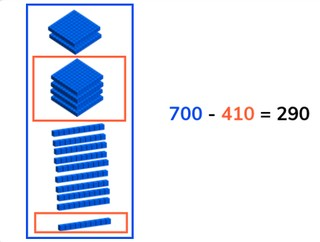Subtraction to 1,000 from a multiple of 100

# Subtraction to 1,000 from a multiple of 100

Learn to subtract numbers to 1,000 from a multiple of 100.

No account needed.8,000 schools use Gynzy92,000 teachers use Gynzy1,600,000 students use Gynzy

## General

Students learn to subtract numbers up to 1,000 from multiples of 100.

## Standards

2.NBT.B.7---!

!---Learning objective
Students will be able to subtract numbers to 1,000 from multiples of 100.

## Introduction

Ask students to solve the difference of the given math problems. Ask students to explain their strategy out loud.

## Development

Explain that you can subtract by counting. You first count how many there are in total, this is the first number of the subtraction problem (the minuend). Then you count how many are taken away, which is the second number of the subtraction problem (the subtrahend). When you take the second from the first you are left with the difference. Show this on the interactive whiteboard using the blocks, and discuss the different problems. This includes problems in which the second number (the subtrahend) has hundreds, tens, and ones. Check that students are able to solve a few problems on their own or in pairs with the given problems with blocks.Ask them to say the problem they are solving out loud, and to say the steps they are taking out loud. Next explain that you can solve these subtraction problems in different ways. Show that you can use a number line to subtract. You can also split the subtrahend and take away the hundreds, tens, and ones in steps. You can also choose to take away the subtrahend in one step. Emphasize to students that they should first carefully look at the problem and then decide which method to use, because all of them work, but some are easier to use on different math problems. Ask students to solve a few problems individually or in pairs, explaining their strategy. Finally walk through the steps of a story problem with the class. Explain that you first determine what kind of math problem it is, then you determine which numbers are important for the problem. You say or write the problem and finally solve the problem. Do one story problem as a class and then ask students to solve another individually or in pairs.

Check that students are able to subtract numbers to 1,000 from a multiple of 100 by asking the following questions:
- What steps do you take to solve a story problem?
- How do you solve 500 - 268?

## Guided Practice

Students are given problems in which they must solve using visual support, solve an abstract problem, and story problems. Encourage students to explain their strategy and walk through the steps of the subtraction problems.

## Closing

Discuss with students that it is important to be able to subtract numbers to 1,000 from a multiple of 100 so that they can easily determine how many they have left (find the difference). Ask students to solve the math problems on the interactive whiteboard. Ask students to explain their strategy. Did they use the same strategy as their neighbor? Have students work in pairs for the final exercise. At the top of the stairs there is a number. As it falls down the stairs it falls apart and parts are taken away. What number is left at the bottom of the stairs?

## Teaching tips

Students who have difficulty with this learning goal can be supported by making use of a number line or other manipulatives. Remind students that they can take away numbers in different steps, start small and then increase the size of the steps as they become more comfortable with subtraction.

## Instruction materials

Optional: MAB blocks or manipulatives, number line

### The online teaching platform for interactive whiteboards and displays in schools

• Save time building lessons

• Manage the classroom more efficiently

• Increase student engagement